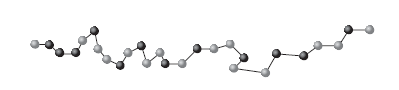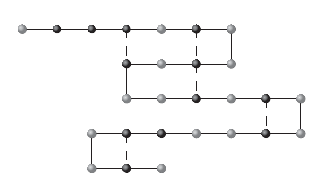# Protein Folding¶

## Objective and Prerequisites¶

Hone your modeling skills with this challenging Protein Folding problem. We’ll show you how to create a binary optimization model of the problem with the Gurobi Python API and then solve it using the Gurobi Optimizer.

This model is example 28 from the fifth edition of Model Building in Mathematical Programming by H. Paul Williams on pages 289-290 and 344-345.

This modeling example is at the advanced level, where we assume that you know Python and the Gurobi Python API and that you have advanced knowledge of building mathematical optimization models. Typically, the objective function and/or constraints of these examples are complex or require advanced features of the Gurobi Python API.

Download the Repository
You can download the repository containing this and other examples by clicking here.

Gurobi License
In order to run this Jupyter Notebook properly, you must have a Gurobi license. If you do not have one, you can request an evaluation license as a commercial user, or download a free license as an academic user.

## Problem Description¶

The problem described in this Jupyter Notebook is based on a molecular biology problem that is discussed in a paper entitled “Quadratic Binary Programming Models in Computational Biology” by Forrester and Greenberg (2008). The problem pertains to a protein, which consists of a chain of amino acids. In this problem, the amino acids come in two forms: hydrophilic (waterloving) and hydrophobic (water hating). An example of such a chain is given in the following figure with the hydrophobic acids marked in bold.Such a chain naturally folds so as to bring as many hydrophobic acids as possible close together. A folding for the chain, in two dimensions, is given in the following figure, with the new matches marked by dashed lines. Our objective is to predict the optimum folding.To solve the problem posed here, we must find the optimum folding for a chain of 50 amino acids with hydrophobic acids at positions 2, 4, 5, 6, 11, 12, 17, 20, 21, 25, 27, 28, 30, 31, 33, 37, 44 and 46.

## Model Formulation¶

### Sets and Indices¶

$k \in A =\{1,2,...,50\}$: Chain of aminoacids.

$i,j \in H =\{2,4,5,6,11,12,17,20,21,25,27,28,30,31,33,37,44,46\} \subseteq Aminoacids$: Subset of aminoacids that are hydrophobic.

### Decision variables¶

$\text{match}_{i,j} \equiv x_{i,j} = 1$, iff hydrophobic acid $i$ is matched with acid $j$, for all hydrophobic acids $i < j \in H$. This matching does not include those matchings that are predefined by virtue of the acids being contiguous in the chain, i.e. $j > i+1$).

$\text{fold}_{k} \equiv y_{k} = 1$, iff a fold occurs between the $i$th and $(i +1)$st acids in the chain.

### Constraints¶

For each pair of hydrophobic acids $i$ and $j$, we can match them if:

• they are not contiguous, that is, already matched,
• they have an even number of acids between them in the chain,
• and there is exactly one fold between $i$ and $j$ .

This gives rise to the following constraints.

1. $y_{k} + x_{i,j} \leq 1, \; \forall k \in A, (i,j) \in H, \; \text{such that} \; i \leq k < j, \; \text{and} \; k \neq (i+j-1)/2$.
2. $x_{i,j} \leq y_{k}, \; \text{where} \; k = (i+j-1)/2$.

Let $\text{H_fold} = \{(i,j) \in H: x_{i,j} \leq y_{k}, \; k = (i+j-1)/2 \}$ be the set of hydrophobic acids for which there is a folding that enables the matching.

### Objective function¶

The objective is to maximize the number of matchings of hydrophobic acids.

$$\sum_{i,j \in \text{H_fold}} x_{i,j}$$

## Python Implementation¶

We import the Gurobi Python Module.

In :
import gurobipy as gp
from gurobipy import GRB

# tested with Python 3.7.0 & Gurobi 9.0


## Input Data¶

In :
# list of aminoacids and hydrophobic acids

acids = [*range(1,51)]

h_phobic = [2,4,5,6,11,12,17,20,21,25,27,28,30,31,33,37,44,46]


## Preprocessing¶

In :
# Creating the data structures to generate the model
list_ij = []

# Indices of hydrophobic acids that can be matched
for i in h_phobic:
for j in h_phobic:
if j > i + 1:
tp = i,j
list_ij.append(tp)

ij = gp.tuplelist(list_ij)

###
list_ik1j = []

list_ik2j = []

for i,j in ij:
for k in range(i,j):
if (k == (i+j-1)/2  ):
tp = i,j,k
list_ik2j.append(tp)
else:
tp = i,j,k
list_ik1j.append(tp)

# Indices for constraints of type 2
ik2j = gp.tuplelist(list_ik2j)

# Indices for constraints of type 1
ik1j = gp.tuplelist(list_ik1j)

# Matchings that are enabled by a folding
list_ijfold = []

for i,j,k in ik2j:
tp = i,j
list_ijfold.append(tp)

ijfold = gp.tuplelist(list_ijfold)


## Model Deployment¶

We create a model and the decision variables. There are two types of decision variables: the variables that determine which hydrophobic acids to match, and the variables that determine at which amino acid the folding of the protein happens.

In :
model = gp.Model('ProteinFolding')

# Matching variables
match = model.addVars(ij, vtype=GRB.BINARY, name="match")

# Folding variables
fold = model.addVars(acids, vtype=GRB.BINARY, name="fold")

Using license file c:\gurobi\gurobi.lic


### Folding and matching constraints¶

1. $y_{k} + x_{i,j} \leq 1, \; \forall k \in A, (i,j) \in H, \; \text{such that} \; i \leq k < j, \; \text{and} \; k \neq (i+j-1)/2$.
2. $x_{i,j} \leq y_{k}, \; \text{where} \; k = (i+j-1)/2$.
In :
# Constraint 1:

C1 = model.addConstrs( (fold[k] + match[i,j] <= 1 for i,j,k in ik1j ) , name='C1')

# Constraint 2:

C2 = model.addConstrs( ( match[i,j] <= fold[k]  for i,j,k in ik2j ) , name='C2')


### Objective function¶

Maximize the matchings of hydrophobic acids.

In :
# Objective function

model.setObjective(gp.quicksum(match[i,j] for i,j in ijfold) , GRB.MAXIMIZE )

In :
# Verify model formulation

model.write('ProteinFolding.lp')

# Run optimization engine

model.optimize()

Gurobi Optimizer version 9.1.0 build v9.1.0rc0 (win64)
Thread count: 4 physical cores, 8 logical processors, using up to 8 threads
Optimize a model with 2441 rows, 197 columns and 4882 nonzeros
Model fingerprint: 0x7a3a8e69
Variable types: 0 continuous, 197 integer (197 binary)
Coefficient statistics:
Matrix range     [1e+00, 1e+00]
Objective range  [1e+00, 1e+00]
Bounds range     [1e+00, 1e+00]
RHS range        [1e+00, 1e+00]
Found heuristic solution: objective -0.0000000
Presolve removed 1596 rows and 105 columns
Presolve time: 0.02s
Presolved: 845 rows, 92 columns, 1779 nonzeros
Variable types: 0 continuous, 92 integer (92 binary)

Root relaxation: objective 3.200000e+01, 90 iterations, 0.00 seconds

Nodes    |    Current Node    |     Objective Bounds      |     Work
Expl Unexpl |  Obj  Depth IntInf | Incumbent    BestBd   Gap | It/Node Time

0     0   32.00000    0   89   -0.00000   32.00000      -     -    0s
H    0     0                       8.0000000   32.00000   300%     -    0s
H    0     0                       9.0000000   32.00000   256%     -    0s
0     0   21.00000    0   69    9.00000   21.00000   133%     -    0s
0     0   13.50000    0   81    9.00000   13.50000  50.0%     -    0s
0     0   12.50000    0   82    9.00000   12.50000  38.9%     -    0s
0     0   12.50000    0   82    9.00000   12.50000  38.9%     -    0s
0     0   12.00000    0   82    9.00000   12.00000  33.3%     -    0s
0     0   12.00000    0   82    9.00000   12.00000  33.3%     -    0s
H    0     0                      10.0000000   11.00000  10.0%     -    0s
0     0   11.00000    0   76   10.00000   11.00000  10.0%     -    0s
0     0   11.00000    0   67   10.00000   11.00000  10.0%     -    0s
0     2   11.00000    0   67   10.00000   11.00000  10.0%     -    0s

Cutting planes:
Gomory: 2
Zero half: 17
RLT: 38

Explored 55 nodes (1222 simplex iterations) in 0.21 seconds
Thread count was 8 (of 8 available processors)

Solution count 4: 10 9 8 -0

Optimal solution found (tolerance 1.00e-04)
Best objective 1.000000000000e+01, best bound 1.000000000000e+01, gap 0.0000%

In :
# Output report

print(f"Optimal number of hydrophobic acids matchings: {model.objVal}")

print("_______________________________________")
print(f"Optimal matching of hydrophobic acids.")
print("_______________________________________")

for i,j,k in ik2j:
if (match[i,j].x > 0.5):
print(f"Hydrophobic acid matching {i,j} with folding at amonacid {k}.")

Optimal number of hydrophobic acids matchings: 10.0
_______________________________________
Optimal matching of hydrophobic acids.
_______________________________________
Hydrophobic acid matching (2, 5) with folding at amonacid 3.
Hydrophobic acid matching (5, 12) with folding at amonacid 8.
Hydrophobic acid matching (6, 11) with folding at amonacid 8.
Hydrophobic acid matching (12, 17) with folding at amonacid 14.
Hydrophobic acid matching (17, 20) with folding at amonacid 18.
Hydrophobic acid matching (20, 25) with folding at amonacid 22.
Hydrophobic acid matching (25, 28) with folding at amonacid 26.
Hydrophobic acid matching (28, 31) with folding at amonacid 29.
Hydrophobic acid matching (31, 46) with folding at amonacid 38.
Hydrophobic acid matching (33, 44) with folding at amonacid 38.


H. Paul Williams, Model Building in Mathematical Programming, fifth edition.

Forrester, R.J. and Greenberg, H.J. (2008) Quadratic Binary Programming Models in Computational Biology. Algorithmic Operations Research, 3, 110–129.

Copyright © 2020 Gurobi Optimization, LLC

In [ ]: